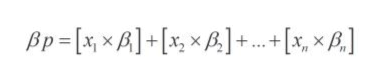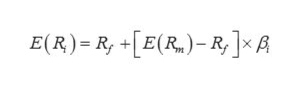Sample bartleby Q&A Solution
You ask questions, our tutors answer
Browse
Question

Illustrate the formula for portfolio beta and portfolio expected return.

## Expert Answer

Portfolio refers to a set of financial investments owned by the investor. The portfolio of investments includes debentures, stocks, bonds, and mutual funds.

Portfolio beta coefficient refers to the systematic risk of a portfolio in relation to the average risky asset in the market. It is the sum of the products of beta coefficients of each asset in the portfolio and their respective weights.

The formula to calculate the portfolio beta:

βP refers to the portfolio beta coefficient

“x1 to xn” refers to the weight of each asset from 1 to “n” in the portfolio

“β1 to βn” refers to the beta coefficient of each asset from 1 to “n” in the portfolio.fullscreen

The formula to calculate the expected return using CAPM:

E(Ri) refers to the expected return on a risky asset

Rf refers to the risk-free rate

E(RM) refers to the expected return on the market portfolio

βi refers to the beta coefficient of the risky asset relative to the market portfoliofullscreen